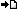RegisterMember LoginForgot Password ?? PHP , ASP , ASP.NET, VB.NET, C#, Java , jQuery , Android , iOS , Windows Phone

## Registered : 108,358

 HOME > PHP Manual > foreach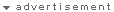## ลองใช้ค้นหาข้อมูล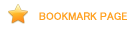## foreach

PHP 4 introduced a foreach construct, much like Perl and some other languages. This simply gives an easy way to iterate over arrays. foreach works only on arrays, and will issue an error when you try to use it on a variable with a different data type or an uninitialized variable. There are two syntaxes; the second is a minor but useful extension of the first:

```foreach (array_expression as \$value)
statement
foreach (array_expression as \$key => \$value)
statement
```

The first form loops over the array given by array_expression. On each loop, the value of the current element is assigned to \$value and the internal array pointer is advanced by one (so on the next loop, you'll be looking at the next element).

The second form does the same thing, except that the current element's key will be assigned to the variable \$key on each loop.

As of PHP 5, it is possible to iterate objects too.

Note: When foreach first starts executing, the internal array pointer is automatically reset to the first element of the array. This means that you do not need to call reset() before a foreach loop.

Note: Unless the array is referenced, foreach operates on a copy of the specified array and not the array itself. foreach has some side effects on the array pointer. Don't rely on the array pointer during or after the foreach without resetting it.

As of PHP 5, you can easily modify array's elements by preceding \$value with &. This will assign reference instead of copying the value.

``` <?php\$arr = array(1, 2, 3, 4);foreach (\$arr as &\$value) {    \$value = \$value * 2;}// \$arr is now array(2, 4, 6, 8)unset(\$value); // break the reference with the last element?> ```
This is possible only if iterated array can be referenced (i.e. is variable).

Warning

Reference of a \$value and the last array element remain even after the foreach loop. It is recommended to destroy it by unset().

Note: foreach does not support the ability to suppress error messages using '@'.

You may have noticed that the following are functionally identical:

``` <?php\$arr = array("one", "two", "three");reset(\$arr);while (list(, \$value) = each(\$arr)) {    echo "Value: \$value<br />\n";}foreach (\$arr as \$value) {    echo "Value: \$value<br />\n";}?> ```
The following are also functionally identical:
``` <?php\$arr = array("one", "two", "three");reset(\$arr);while (list(\$key, \$value) = each(\$arr)) {    echo "Key: \$key; Value: \$value<br />\n";}foreach (\$arr as \$key => \$value) {    echo "Key: \$key; Value: \$value<br />\n";}?> ```

Some more examples to demonstrate usages:

``` <?php/* foreach example 1: value only */\$a = array(1, 2, 3, 17);foreach (\$a as \$v) {   echo "Current value of \\$a: \$v.\n";}/* foreach example 2: value (with its manual access notation printed for illustration) */\$a = array(1, 2, 3, 17);\$i = 0; /* for illustrative purposes only */foreach (\$a as \$v) {    echo "\\$a[\$i] => \$v.\n";    \$i++;}/* foreach example 3: key and value */\$a = array(    "one" => 1,    "two" => 2,    "three" => 3,    "seventeen" => 17);foreach (\$a as \$k => \$v) {    echo "\\$a[\$k] => \$v.\n";}/* foreach example 4: multi-dimensional arrays */\$a = array();\$a = "a";\$a = "b";\$a = "y";\$a = "z";foreach (\$a as \$v1) {    foreach (\$v1 as \$v2) {        echo "\$v2\n";    }}/* foreach example 5: dynamic arrays */foreach (array(1, 2, 3, 4, 5) as \$v) {    echo "\$v\n";}?> ```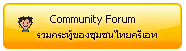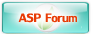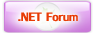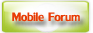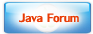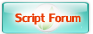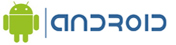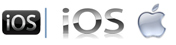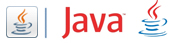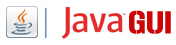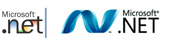Free Tutorial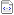สอน Google Maps Apiสอน Windows Serviceสอน Entity Frameworkสอน Androidสอน Java เขียน JavaJava GUI Swingสอน JSP (Web App)iOS (iPhone,iPad)Windows PhoneWindows AzureWindows StoreLaravel FrameworkYii PHP Frameworkสอน jQueryสอน jQuery กับ Ajaxสอน PHP OOP (Vdo)Ajax Tutorials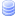SQL Tutorialsสอน SQL (Part 2)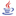JavaScript TutorialJavascript TipsVBScript TutorialVBScript ValidationMicrosoft Access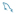MySQL Tutorials-- Stored ProcedureMariaDB Database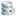SQL Server TutorialSQL Server 2005SQL Server 2008SQL Server 2012-- Stored ProcedureOracle Database-- Stored ProcedureSVN (Subversion)แนวทางการทำ SEOปรับแต่งเว็บให้โหลดเร็วสุดยอด Source Code V2.0แจ้งชำระเงิน/โอนเงินลองค้นหาสิ่งที่ต้องการLoad balance : Server 03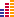© www.ThaiCreate.Com. 2003-2020 All Rights Reserved. ไทยครีเอทบริการ จัดทำดูแลแก้ไข Web Application ทุกรูปแบบ (PHP, .Net Application, VB.Net, C#) [Conditions Privacy Statement] ติดต่อโฆษณา 081-987-6107 อัตราราคา คลิกที่นี่